# Resources tagged with: Fractions

Filter by: Content type:
Age range:
Challenge level:

### There are 64 results

Broad Topics > Fractions, Decimals, Percentages, Ratio and Proportion > Fractions### A Chance to Win?

##### Age 11 to 14 Challenge Level:

Imagine you were given the chance to win some money... and imagine you had nothing to lose...### Fractions Rectangle

##### Age 11 to 14 Challenge Level:

The large rectangle is divided into a series of smaller quadrilaterals and triangles. Can you untangle what fractional part is represented by each of the ten numbered shapes?### Pythagoras’ Comma

##### Age 14 to 16 Challenge Level:

Using an understanding that 1:2 and 2:3 were good ratios, start with a length and keep reducing it to 2/3 of itself. Each time that took the length under 1/2 they doubled it to get back within range.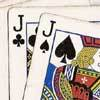### Do Unto Caesar

##### Age 11 to 14 Challenge Level:

At the beginning of the night three poker players; Alan, Bernie and Craig had money in the ratios 7 : 6 : 5. At the end of the night the ratio was 6 : 5 : 4. One of them won \$1 200. What were the. . . .### Racing Odds

##### Age 11 to 14 Challenge Level:

In a race the odds are: 2 to 1 against the rhinoceros winning and 3 to 2 against the hippopotamus winning. What are the odds against the elephant winning if the race is fair?### Circuit Training

##### Age 14 to 16 Challenge Level:

Mike and Monisha meet at the race track, which is 400m round. Just to make a point, Mike runs anticlockwise whilst Monisha runs clockwise. Where will they meet on their way around and will they ever. . . .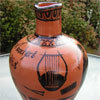### Six Notes All Nice Ratios

##### Age 14 to 16 Challenge Level:

The Pythagoreans noticed that nice simple ratios of string length made nice sounds together.### F'arc'tion

##### Age 14 to 16 Short Challenge Level:

At the corner of the cube circular arcs are drawn and the area enclosed shaded. What fraction of the surface area of the cube is shaded? Try working out the answer without recourse to pencil and. . . .### Equal Temperament

##### Age 14 to 16 Challenge Level:

The scale on a piano does something clever : the ratio (interval) between any adjacent points on the scale is equal. If you play any note, twelve points higher will be exactly an octave on.### Bull's Eye

##### Age 11 to 14 Challenge Level:

What fractions of the largest circle are the two shaded regions?### Plutarch's Boxes

##### Age 11 to 14 Challenge Level:

According to Plutarch, the Greeks found all the rectangles with integer sides, whose areas are equal to their perimeters. Can you find them? What rectangular boxes, with integer sides, have. . . .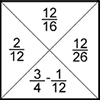### Fractions Jigsaw

##### Age 11 to 14 Challenge Level:

A jigsaw where pieces only go together if the fractions are equivalent.### Smaller and Smaller

##### Age 7 to 14 Challenge Level:

Can you predict, without drawing, what the perimeter of the next shape in this pattern will be if we continue drawing them in the same way?### Farey Sequences

##### Age 11 to 14 Challenge Level:

There are lots of ideas to explore in these sequences of ordered fractions.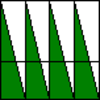### Teaching Fractions with Understanding: Part-whole Concept

##### Age 5 to 14

Written for teachers, this article describes four basic approaches children use in understanding fractions as equal parts of a whole.### Water Lilies

##### Age 11 to 14 Challenge Level:

There are some water lilies in a lake. The area that they cover doubles in size every day. After 17 days the whole lake is covered. How long did it take them to cover half the lake?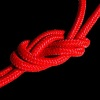### Twisting and Turning

##### Age 11 to 14 Challenge Level:

Take a look at the video and try to find a sequence of moves that will untangle the ropes.### Investigating the Dilution Series

##### Age 14 to 16 Challenge Level:

Which dilutions can you make using only 10ml pipettes?### The Genes of Gilgamesh

##### Age 14 to 16 Challenge Level:

Can you work out the parentage of the ancient hero Gilgamesh?##### Age 11 to 14 Challenge Level:

Can you work out which drink has the stronger flavour?### Fractions and Percentages Card Game

##### Age 11 to 16 Challenge Level:

Can you find the pairs that represent the same amount of money?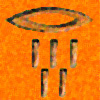### History of Fractions

##### Age 7 to 14

Who first used fractions? Were they always written in the same way? How did fractions reach us here? These are the sorts of questions which this article will answer for you.##### Age 11 to 14 Challenge Level:

Aisha's division and subtraction calculations both gave the same answer! Can you find some more examples?### Couples

##### Age 11 to 14 Challenge Level:

In a certain community two thirds of the adult men are married to three quarters of the adult women. How many adults would there be in the smallest community of this type?### Fair Shares?

##### Age 14 to 16 Challenge Level:

A mother wants to share a sum of money by giving each of her children in turn a lump sum plus a fraction of the remainder. How can she do this in order to share the money out equally?### Tiny Nines

##### Age 14 to 16 Challenge Level:

What do you notice about these families of recurring decimals?### Tweedle Dum and Tweedle Dee

##### Age 11 to 14 Challenge Level:

Two brothers were left some money, amounting to an exact number of pounds, to divide between them. DEE undertook the division. "But your heap is larger than mine!" cried DUM...### Hello Again

##### Age 11 to 14 Challenge Level:

Anne completes a circuit around a circular track in 40 seconds. Brenda runs in the opposite direction and meets Anne every 15 seconds. How long does it take Brenda to run around the track?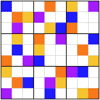### Ratio Sudoku 3

##### Age 11 to 16 Challenge Level:

A Sudoku with clues as ratios or fractions.### Almost One

##### Age 11 to 14 Challenge Level:

Choose some fractions and add them together. Can you get close to 1?### Unit Fractions

##### Age 11 to 14 Challenge Level:

Consider the equation 1/a + 1/b + 1/c = 1 where a, b and c are natural numbers and 0 < a < b < c. Prove that there is only one set of values which satisfy this equation.### How Long Is the Cantor Set?

##### Age 11 to 14 Challenge Level:

Take a line segment of length 1. Remove the middle third. Remove the middle thirds of what you have left. Repeat infinitely many times, and you have the Cantor Set. Can you find its length?### Harmonic Triangle

##### Age 14 to 16 Challenge Level:

Can you see how to build a harmonic triangle? Can you work out the next two rows?### Counting Fish

##### Age 14 to 16 Challenge Level:

I need a figure for the fish population in a lake. How does it help to catch and mark 40 fish?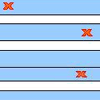### Stretching Fractions

##### Age 14 to 16 Challenge Level:

Imagine a strip with a mark somewhere along it. Fold it in the middle so that the bottom reaches back to the top. Stetch it out to match the original length. Now where's the mark?### Archimedes and Numerical Roots

##### Age 14 to 16 Challenge Level:

The problem is how did Archimedes calculate the lengths of the sides of the polygons which needed him to be able to calculate square roots?### More Twisting and Turning

##### Age 11 to 16 Challenge Level:

It would be nice to have a strategy for disentangling any tangled ropes...### The Greedy Algorithm

##### Age 11 to 14 Challenge Level:

The Egyptians expressed all fractions as the sum of different unit fractions. The Greedy Algorithm might provide us with an efficient way of doing this.### Tangles

##### Age 11 to 16

A personal investigation of Conway's Rational Tangles. What were the interesting questions that needed to be asked, and where did they lead?### Peaches Today, Peaches Tomorrow...

##### Age 11 to 14 Challenge Level:

A monkey with peaches, keeps a fraction of them each day, gives the rest away, and then eats one. How long can his peaches last?### Rod Fractions

##### Age 7 to 14 Challenge Level:

Pick two rods of different colours. Given an unlimited supply of rods of each of the two colours, how can we work out what fraction the shorter rod is of the longer one?### Rationals Between...

##### Age 14 to 16 Challenge Level:

What fractions can you find between the square roots of 65 and 67?### Fracmax

##### Age 14 to 16 Challenge Level:

Find the maximum value of 1/p + 1/q + 1/r where this sum is less than 1 and p, q, and r are positive integers.### Countdown Fractions

##### Age 11 to 16 Challenge Level:

Here is a chance to play a fractions version of the classic Countdown Game.### Stretching Fractions: A Discussion

##### Age 14 to 18

This article extends and investigates the ideas in the problem "Stretching Fractions".### Terminating or Not

##### Age 11 to 14 Challenge Level:

Is there a quick way to work out whether a fraction terminates or recurs when you write it as a decimal?### Matching Fractions, Decimals and Percentages

##### Age 7 to 14 Challenge Level:

Can you match pairs of fractions, decimals and percentages, and beat your previous scores?### Special Sums and Products

##### Age 11 to 14 Challenge Level:

Find some examples of pairs of numbers such that their sum is a factor of their product. eg. 4 + 12 = 16 and 4 × 12 = 48 and 16 is a factor of 48.### Sum Equals Product

##### Age 11 to 14 Challenge Level:

The sum of the numbers 4 and 1 [1/3] is the same as the product of 4 and 1 [1/3]; that is to say 4 + 1 [1/3] = 4 � 1 [1/3]. What other numbers have the sum equal to the product and can this be so. . . .### Lower Bound

##### Age 14 to 16 Challenge Level:

What would you get if you continued this sequence of fraction sums? 1/2 + 2/1 = 2/3 + 3/2 = 3/4 + 4/3 =